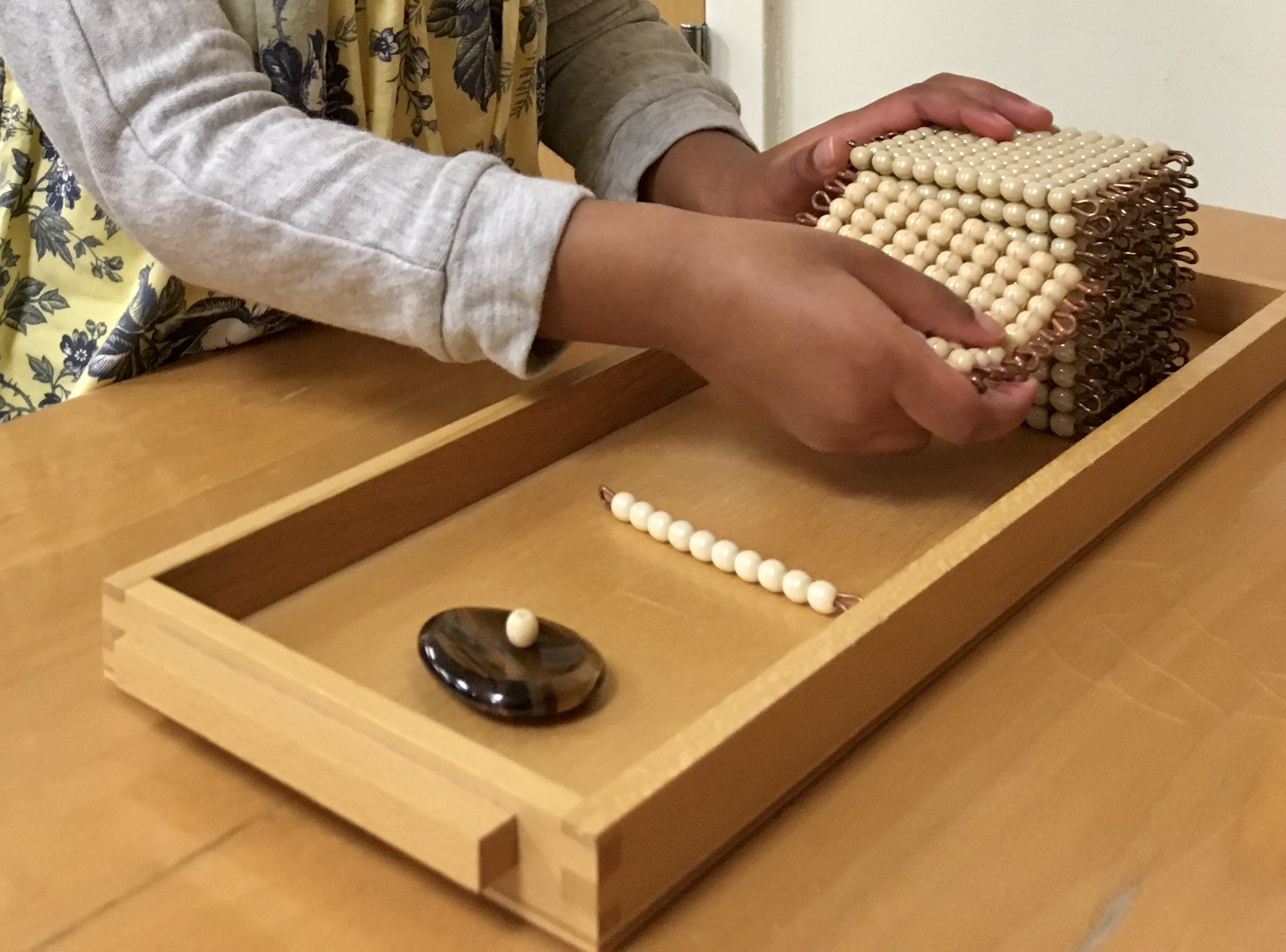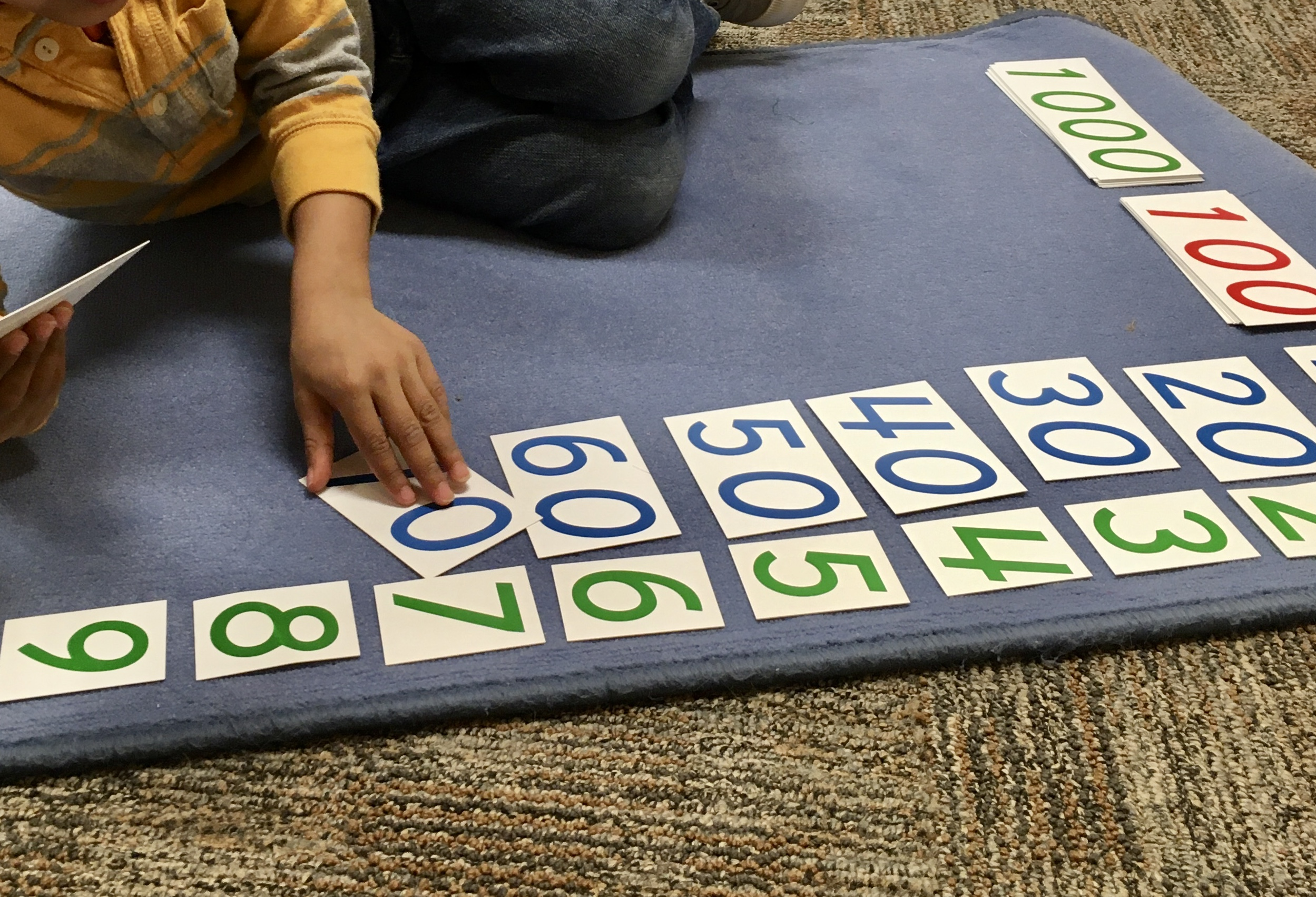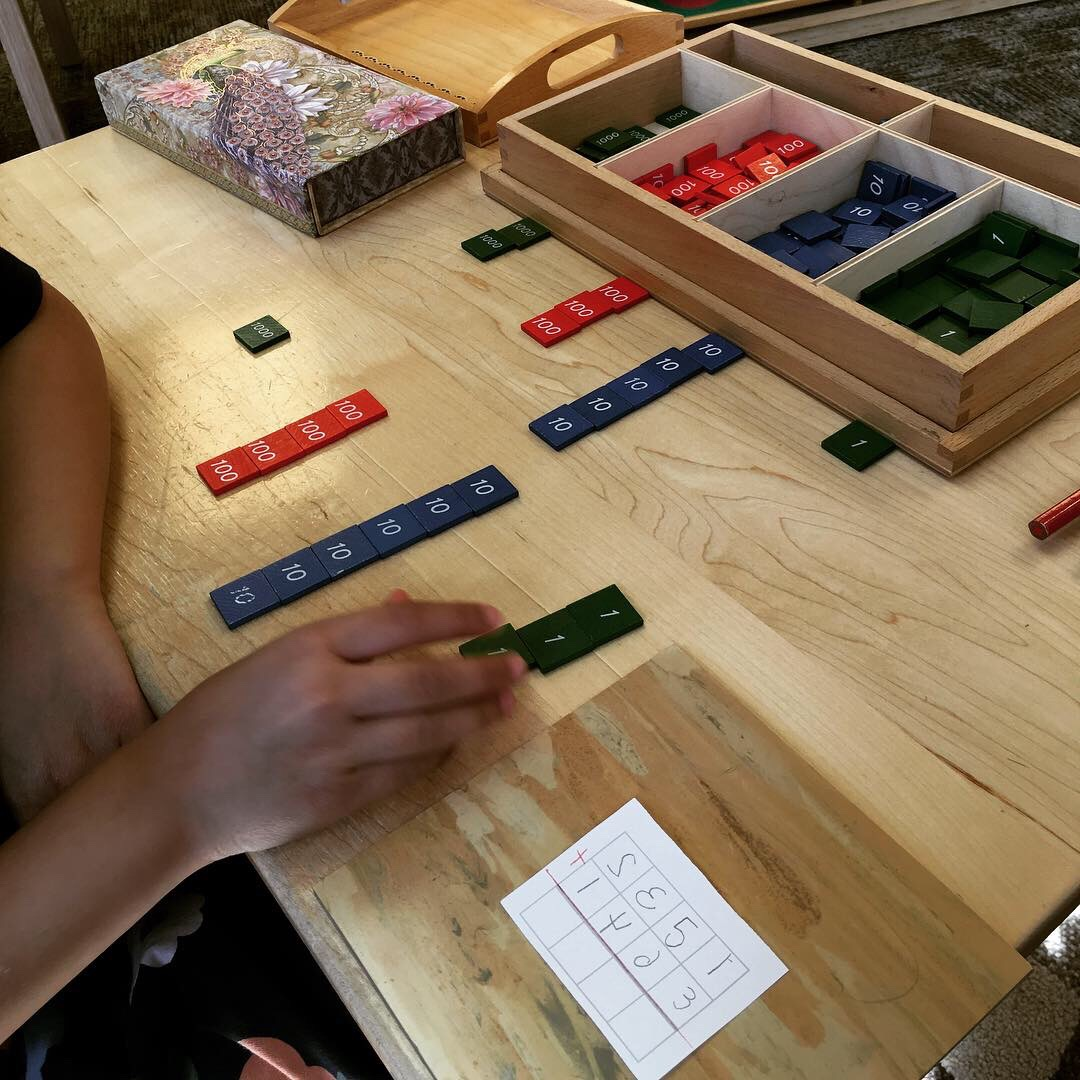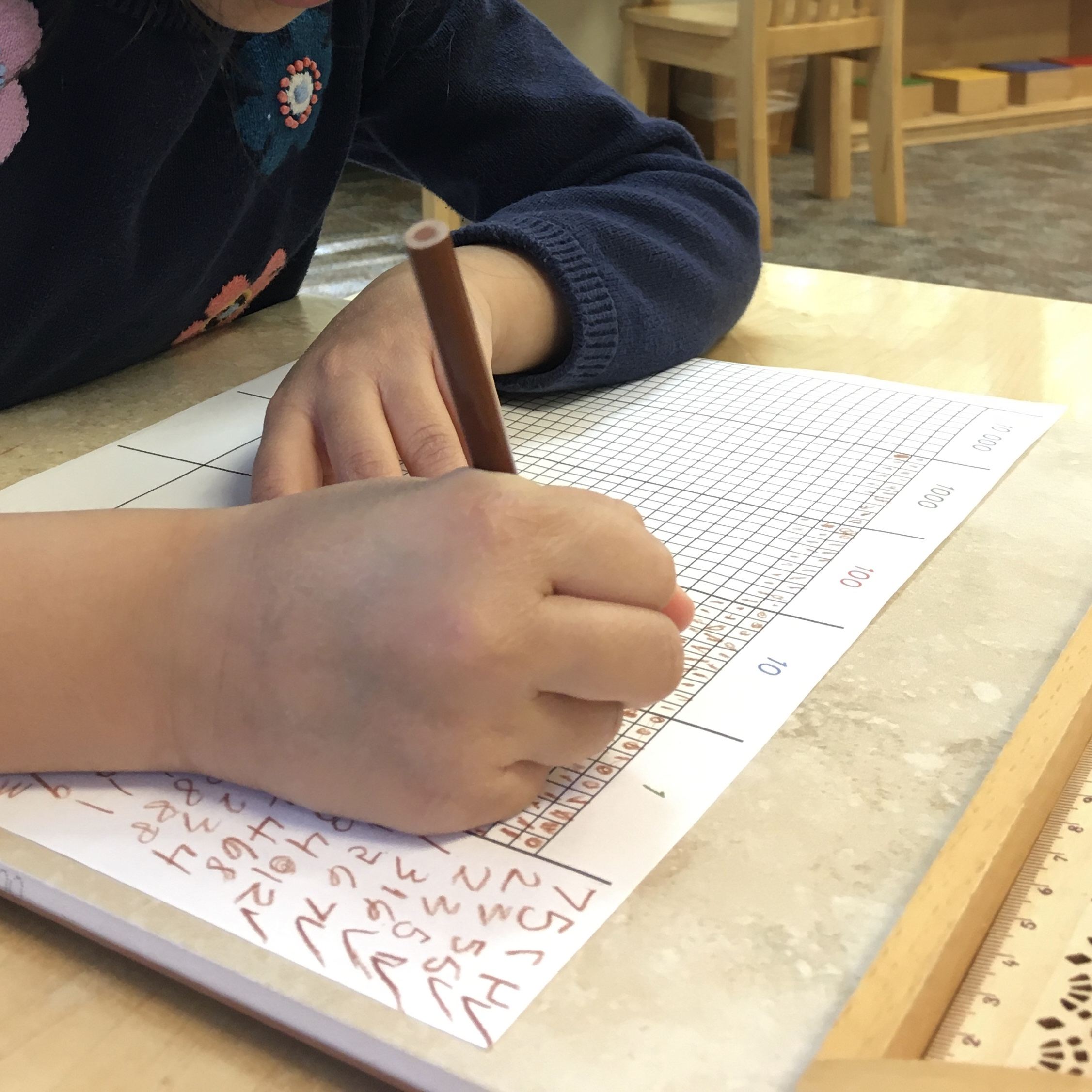# The Decimal System

Explore activities that introduce the decimal system and the four operations.These activities introduce the child to the function of our base-ten number system and the four operations: addition, subtractions, multiplication, and division. As with all of the Montessori math materials, these lessons are introduced using hands-on materials. We use the golden bead material to introduce one unit, one ten, one hundred, and one thousand, and allow the child to see and feel the relative difference between the quantities. We then introduce the numeric symbols using the decimal system cards. The child then sees that these beads and symbols together form the foundation of our number system. We also use the golden bead material to introduce the children to the four operations. They experience putting numbers together through addition, taking beads away through subtraction, combining the same number of beads many times for multiplication, and sharing a quantity equally through division. The child can practice these operations in a group through the collective exercises of golden beads or alone using the stamp game and the dot game.

## At Home Decimal System Activities:

### Presentation with Beads  ages 4 ½ and up

Notes: The children are introduced to one unit, one ten, one hundred, and one thousand using concrete materials. Here is a diagram of the material that can be printed and reviewed.

### Presentation with Cards  ages 4 ½ and up

Notes: The children are introduced to the numeric representations of one unit, one ten, one hundred, and one thousand using the decimal system cards. Here is a diagram of the material that can be printed and reviewed.

### Decimal System Beads  ages 4 ½ and up

Directions: Print and cut out the pictures of the units, tens, hundreds, and thousands for decimal system lessons and additional practice.

### “Bring Me” Games with Decimal System Beads  ages 4 ½ and up

Directions: “Bring Me” games are a fun way to help children practice building quantities with the decimal system beads. Have your child lay out all of their decimal system beads, placing the units together, the tens together, the hundreds together, and the thousands together. Ask the child to bring quantities from one, two, three, or four categories based on the child’s ability. For more information, refer to the Bring Me Games with the Decimal System Beads-Corrected document.### Decimal System Cards  ages 4 ½ and up

Directions: Print and cut out the cards for decimal system lessons and additional practice.

### “Bring Me” Games with the Decimal System Cards  ages 4 ½ and up

Directions: “Bring Me” games are a great way to help children practice forming numbers with the decimal system cards. Have your child lay out the decimal system cards, including the units, tens, hundreds, and thousands. Ask the child to bring cards from one, two, three, or four categories based on the child’s ability. For more information, download the Bring Me Games with the Decimal System.### The Stamp Game  ages 5 to 6 ½

Notes: The Stamp Game offers children the opportunity to explore addition, subtraction, multiplication, and division all by themselves. By working with the stamp game, children learn how to compose numbers, write their own equations, and are introduced to the various operation signs. These concepts are introduced gradually by the teacher so that the child has time to practice and master each operation.

### Stamp Game Equations  ages 5 to 6 ½

Notes: In the classroom, we strongly encourage children to write their own equations. However, we often write the first few problems to give the children an example of how to write appropriate equations. Here are a few sample equations for each operation.### The Dot Game  ages 5 - 6

Notes: The Dot Game offers children the opportunity to explore the addition of multiple addends. Typically, with golden beads or the stamp game, children put together two or three numbers. With the dot game, children can put together as many numbers as they can fit on the paper.